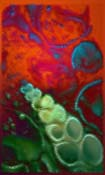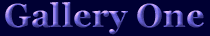Click on image to enjoy a larger Earth Art image with MIDI music.

E  a  r  t  h    L  i g  h  t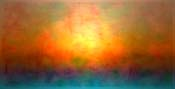T  h  e    S  e  e  d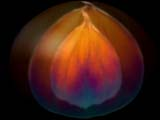A  u  r  o  r  a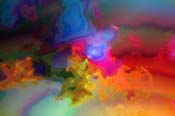H  e  a  r  t   S  o  u  r  c  eB  r  o  o  k  f  i  e  l  d   F  e  r  n  s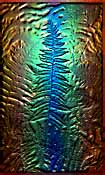R  e  m  e  m  b  r  a  n  c  e  s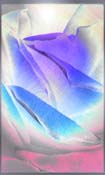S  k  y   Y  e  a  r  n  i  n  g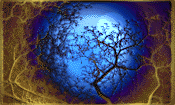T  h  o  u  g  h  t  s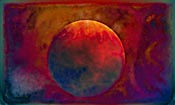E  a  r  t  h   A  l  p  h  aT  h  e   S  h  e  l  l